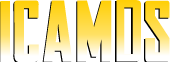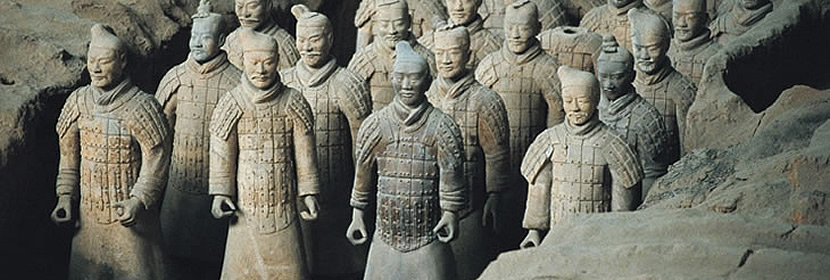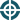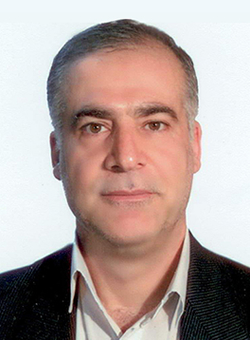View More Conferences 更多会议 中文版2017 2nd International Conference on Applied Mathematics and Data Science
Apr. 27-28, 2017, Xi’an, ChinaBecome SpeakersPaper SubmissionJoin as ReviewersJoin CommitteeKeynote Speakers
The information about the Keynote Speakers of ICAMDS2017 is as follows, which will be updated regularly.Dr. Alaeddin Malek, Associate Professor
Department of Applied Mathematics, Faculty of Mathematical Sciences, Tarbiat Modares University, Iran

Biography: Alaeddin Malek received his M.Sc. and Ph.D. degrees in Applied Mathematics from McGill University, Canada, and University of Wales, UK, in years 1986 and 1991. At the moment he is an Associate Professor in Department of Applied Mathematics, Tarbiat Modares University, Tehran, Iran. His current research interests include Neural networks, Optimization and control theory, Dynamical systems, Heat transformation, Thin films, Heat management in composites, Applications of mathematics in biomedical engineering and Numerical solutions for PDEs. He is the co-author in the 21 books written in the Persian language. He has published more than 48 papers in the international journals.He was keynote speaker for the International Conference of Mathematical Sciences, (2009), Istanbul, Turkey, the 4th Iranian Conference on Numerical Analysis and its Applications, (2013), the 22nd Iranian Conference on Biomedical Engineering (2015) and Second National Conference on Computational Sciences (2016). His talk entitled “Optimization with Recurrent Neural Networks: Recent Advances and New Perspectives”, “Synchronization Control for Flying Robots Formation”, “Application of Mathematics in Biomedical Engineering” and “Solving Convection Equation in Porous Area using Spectral Methods” respectively.

Topic: Efficient Techniques for Free Convection in an Inclined Saturated Porous Medium

Abstract: The physical process for heat transfer in fluid-saturated porous medium contains both the mass conservation (continuity equation) and the energy conservation (Darcy’s law and the heat transfer equation). Some numerical codes that have potential to solve simple types of this problem are: ANSYS FLUENT, Sutrasoftware, Wolfram Mathematica 10, Open FOAM the open source CFD Toolbox and COMSOL MULTIPHYSICS. In this talk, I focus on proposing the semi-analytic Fourier-Galerkin solution to the problem, while the finite element solution is given for the evaluation of the proposed method. This talk is organized as follows: First; the problem geometry is defined as an inclined square porous cavity whose four walls are maintained at different temperatures or heat fluxes and the horizontal walls are assumed to be adiabatic. Second; the problem is modeled and solved semi-analytically using the Fourier-Galerkin method by (i) Applying a rotation transformation to rewrite the equation in the transformed system, (ii) Reformulating the governing equation using the stream function and the temperature as unknowns, (iii) Expanding the unknowns in appropriate Fourier series, (iv) Substituting the series in the novel governing equation (v) Treating the resulting equation by using the Galerkin technique, (vi) Solving the final system to get the Fourier coefficients. Third; Numerical solution is performed by using the Free Convection in Porous Media package (FCPM) that is developed by the LHyGes institute at Strasbourg University. FCPM combines specific numerical methods for time integration and spatial discretization to efficiently solve the equations of free convection in porous media. Comparisons are made between the solutions to show the efficiency of the proposed method.

In Chinese >>